## Pages from a Physicist's Notebook

In this series, aimed at police collision analysts and reconstructionists, the author derives some of the equations used in motor vehicle collision reconstruction from first principles. Certain methods are illustrated with real-world examples, and various computer-based solutions are discussed. Brief descriptions of the topics covered in the present series are provided below, together with links for downloading the individual articles.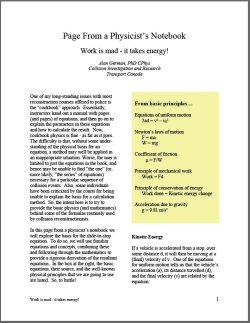Work is mad - it takes energy! In this page from a physicist’s notebook we will explore the basis for the slide-to-stop equation. To do so, we will use familiar equations and concepts, combining these and following through the mathematics to provide a rigorous derivation of the resultant equations. The paper develops the equation for kinetic energy and applies this to fully-locked braking to a stop, the so-called slide-to-stop. Braking over multiple surfaces and the physics behind the "combined speed equation" are also discussed. An example of the application of the principle of conservation of energy to a crash involving pre-impact braking is given to illustrate a more general use of combining energies over a series of collision events.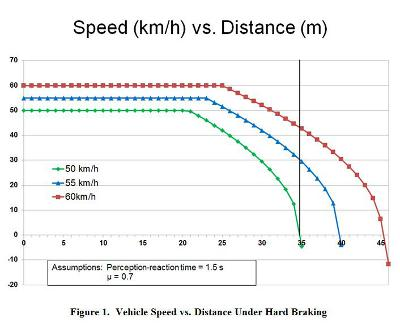Stopping on a dime - or perhaps on a loonie! Following up on the concepts used to determine that "work is mad", we explore the possibilities for vehicles to "stop on a dime" and discover that, sometimes, they actually need a loonie! The paper provides an example of calculating a vehicle's total stopping distance as the distance travelled during the period of the driver's perception-reaction to a hazard and the distance travelled under subsequent hard braking. Similar calculations for different initial speeds show the potential dangers in increased speed. We look at a vehicle that can just brake to a halt and avoid a crash and find that a vehicle travelling just 5 km/h faster will undergo a collision with an impact speed of 30 km/h!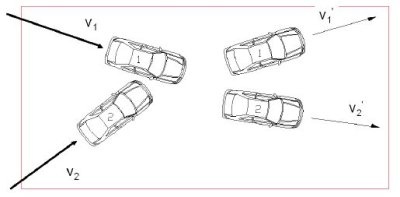Momentum 101 - The principle of conservation of linear momentum For police officers, the principle of the conservation of linear momentum is the essence of Level IV training for collision reconstructionists. While courses on this topic are often focused on the individual parameters in the momentum equation, and how to use the equation to calculate vehicle speeds, the actual science behind the process is sometimes not well understood. In this page from a physicist’s notebook we will explore familiar equations and concepts, and combine these with the appropriate mathematics, to develop the basic equation governing the conservation of linear momentum.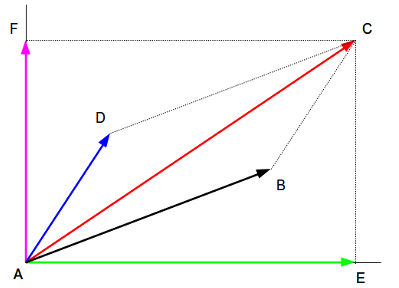Momentum 102 - Vector Analysis and Momentum Because momentum is the product of a scalar quantity (vehicle mass) and a vector quantity (vehicle velocity), momentum itself is a vector. And, since both the magnitude (size) and direction of vector quantities are important, working with this equation requires the application of vector analysis rather than simple algebra. In this page from a physicist’s notebook, we will look at the properties of vectors, how to add them together to determine a resultant vector, and, in particular, how to find the initial speed of each of two vehicles involved in a vehicle-to-vehicle crash.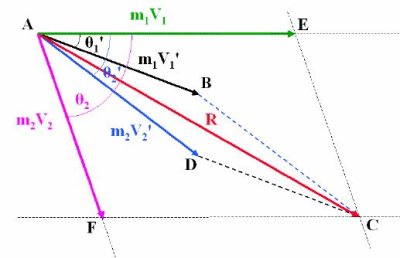Momentum 103 - The algebraic solution for conservation of momentum In the first article in the series we developed the equation for the conservation of linear momentum. The second article implemented a graphical solution to the equation for the conservation of linear momentum. In particular, we used a vector diagram, drawn to scale, to obtain the solution for the initial speeds of two-vehicles involved in an angled collision. In the current article, we will consider a general form of the vector diagram for a two-vehicle collision, and develop a purely algebraic solution. We will also see how this can be programmed as a computer spreadsheet to rapidly provide a solution for a specific set of input parameters.Falls and Vaults – These really are rocket science! Normally, the catch phrase for the physics and mathematics that are necessary to perform calculations related to motor vehicle collisions is that “It's not rocket science!” However, the subject of falls and vaults is – more-or-less – rocket science. They both involve consideration of a projectile (a vehicle, an occupant – or a motorcycle rider) being launched into the air and following a free-flight trajectory under the action of gravity. We generally want to calculate the speed at take-off based on the angle of projection, and the horizontal and vertical distances travelled between the points of take-off and landing. As usual, in our Page from a Physicist’s Notebook, we will use physics and mathematics obtained from first principles to derive the equations that can be applied directly to motor vehicle crashes involving falls and vaults. Case studies taken from real-world collisions are used as examples of the calculation methodologies In addition, a simple computer spreadsheet that can be used to solve the general vault equation is described, as is a graphical method where a series of x-y trajectories are plotted for a range of take-off speeds and a specific angle of projection. Also available is a spreadsheet that will perform the speed calculation derived in the paper. The spreadsheet is in Microsoft Excel 97-2003 workbook format (XLS) and will work in either Excel or in OpenOffice.org Calc.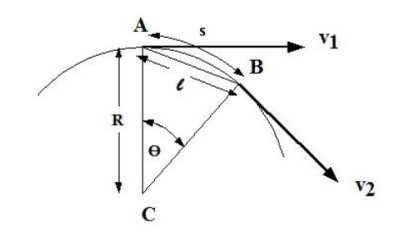Yaw – Going round in circles! In this Page from a Physicist’s Notebook, we will take a look at the physics involved in motion in a circle. We will explore the difference between speed and velocity, and see how these apply to a vehicle travelling at constant speed around a circular path. We will use physics and mathematics obtained from first principles to derive an equation for the vehicle's lateral acceleration, and to quantify the frictional side force that is necessary to maintain circular motion. These relationships will allow us to derive the equation that can be used to calculate the critical vehicle speed for a curve. This article was first developed for a motorcycle collision investigation course and so a discussion of lean angle is also included.

 Road Safety is Everyone's Responsibility Are you part of the problem - or part of the solution?